# Kerala Syllabus 9th Standard Chemistry Solutions Chapter 3 Redox Reactions and Rate of Chemical Reactions

You can Download Redox Reactions and Rate of Chemical Reactions Questions and Answers, Summary, Activity, Notes, Kerala Syllabus 9th Standard Chemistry Solutions Part 1 Chapter 3 help you to revise complete Syllabus and score more marks in your examinations.

## Kerala State Syllabus 9th Standard Chemistry Solutions Chapter 3 Redox Reactions and Rate of Chemical Reactions

### Redox Reactions and Rate of Chemical Reactions Textual Questions and Answers

Activity -1

Given below is a table showing the mass of the reactants and the products when hydrogen combines with chlorine to form hydrogen chloride in two situations.HSSlive chemistry redox reactions Question 1.
Write down the total mass of the reactants and also the total mass of product in the above experiment.
a) Situation 1: …………………
b) Situation 2: ……………….
a) Total mass of reactants 73g products 73g
b) Reactants 146g products 146 gActivity-2

Given below is a table showing the mass of the reactants and the products when hydrogen combines with oxygen to form water, in two situations.what is redox reaction class 10 Question 2.
Write down the total mass of the reactants and the total mass of product in the above experiment.
a) Situation 1:
b) Situation 2:
a) Reactants 18 g, Product 18 g
b) Reactants 36 g, Products 36 g

Question 3.
What is the relation between the total mass of the reactants and the total mass of products?
Both are equal.

State The Law Of Conservation Of Mass

In a chemical reaction mass is neither created nor destroyed. This is the law of conservation of mass.
This law was proposed by the scientist Antoine Lavoisier. That is, the total mass of the reactants will be equal to the total mass of the products.

Activity-3

redox reaction and rate of chemical reaction Question 4.
A piece of magnesium is burned in air. What do you observe?
It burns with sparkling light

Name the reducing agent in given below chemical reaction Question 5.
What is the white powder formed?
Magnesium oxide (MgO)

Question 6.
Which are the reactants here?
Mg and O2

chemical reaction equalizer Question 7.
Which is the product?
MgO

redox reactions questions and answers Question 8.
Note down the number of atoms in the reactant side and the number of atoms in the product side in the table below.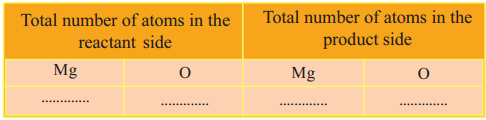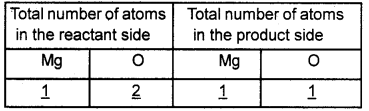Question 9.
Is the number of atoms of each element equal on both sides?
No

Question 10.
The number of which atom is not equal on both sides?
O

Question 11.
How many product molecules are needed to equalize the number of oxygen atoms on both sides?
2

Question 12.
How will you represent two molecules of magnesium oxide?
2 MgO

Question 13.
Now, is the number of magnesium atoms equal on both sides?
No

Question 14.
How many magnesium atoms are needed in the reactant side to equalize the number of magnesium atoms on both sides?
2

Question 15.
Then how can you rewrite the above equation?
2 Mg + O2 2MgO

Question 16.
Is the total number of atoms of each element in the molecules present in the reactant side and that in the product side equal in this equation?
YesQuestion 17.
What is balancing of equations?
Equalizing the number of atoms of each element in molecules in the reactant side and that in the product side is called balancing of equation.
Zn + HCl → ZnCl2 + H2
Tabulate the total number of atoms of each elements in the reactant side and that in the product side of the above reaction.Question 18.
How many Zn atoms are there in the reactant side and in the product side?
1 each

Question 19.
Which are the atoms showing a difference in their number?
H and Cl

Question 20.
In order to make them equal on both sides how many molecules of HCI should be taken as reactant.
2
Now rewrite the equation
Zn + HCl → ZnCl2 + H2

Question 21.
Balance the chemical equation H2 + O2 → H2O
Step I: H2+ O2 → H2O
Step II: 2H2 + O2 → 2H2O

Question 22.
Balance the chemical equation Al + O2 → Al2O2
Step I: 2Al + O2 → Al2O3
Step II: 2Al+ 3O2 → 2Al2O3

Question 23.
Balance the chemical equation H2 + O2 → H2O
Step I: H2 + O2 → 2H2O
Step II: 2H2 + O2 → 2H2O

Question 24.
Some chemical equations are given below. Note down the number of reactant atoms and that of product atoms in the table given below.
1. C + O2 → CO2
2. N2 + H2 → NH3
3. 2H2 O2 → 2H2O + O2
4. SO2 + O2 → SO3
5. BaCl2 + H2SO4 → BaSO+2HCl

 No. Reactant atoms Product atoms 1 C-1, O-2 C-1, O-2 2 N-2, H-2 N-1,  H-3 3 H-4, O-4 H-4, O-4 4. S-1, O-4 S-1, O-3 5. H-2, S-1, O-4, H-2, S-1,O-4, Ba-1 Cl-2 Ba-1, Cl-2

Balance the equations which are unbalanced
1. N2 + 3H2 → 2NH3
2. 2SO2 + O2 → 2SO3

Oxidation And Reduction

Question 25.
The electronic configuration of magnesium and chlorine are 2, 8, 2 and 2, 8, 7 respectively. How many electrons does a magnesium atom donate? What charge will it get?
2, two positive (2+)

Question 26.
Let us complete the equation for this process,
Mg → Mg2+ + ……………
Mg → Mg2+ + 2e

Question 27.
How many electrons are accepted by each chlorine atom? What will be the charge acquired by each atom?
One 1 Cl1-

Question 28.
Complete the equation of this process.
Cl + 1e
Cl + 1e → Cl1-

Question 29.
What are oxidation and reduction?
Oxidation is the process of loss of electrons. Reduction is the process of gain of electrons. The atom which loss electron is called the reducing agent and the atom which gains electron is called the oxidizing agent.

Question 30.
In the above chemical reaction, which atom is oxidized?
Sodium

Question 31.
Which atom is reduced?
Chlorine

Question 32.
Which is the oxidizing agent in this chemical reaction?
Chlorine (Cl)

Question 33.
Which is the reducing agent in this chemical reaction?
Sodium (Na)

Question 34.
Analyze the following equations and list the oxidized atom, reduced atom, oxidizing agent and reducing agent.
a) Mg + F2 → MgF2
b) 2Na + Cl → NaCl
a) Oxidized atom — Mg
Equation of oxidation — Mg → Mg2+ + 2e
Reduced atom — F
Equation of reduction — F + e → F
Oxidizing agent — F
Reducing agent — Mg

b) Oxidized atom — Na
Equation of oxidation — Na → Na + 1e
Reduced atom — Cl
Equation of reduction — Cl+ 1e → Cl
Oxidizing agent — Na
Reducing agent — Cl

Question 35.
Analyse the following equations and complete the Table given below.
1. Mg → Mg2+ + 2e
F+ le → F
2. Na → Na++1e
Cl + 1e → Cl
3. Fe → Fe2++ 2e
O + 2e → O2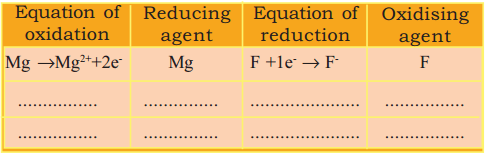Equation of oxidation Reducing agent Equation of reduction Oxidizing agent Mg → Mg2++ 2e– Mg F + 1e–→ F– F Na → Na++1e– Na Cl + 1e → Cl–Cl Cl Fe → Fe2++ 2e– Fe O + 2e– → O2- O

Oxidation Number

Oxidation number of an atom in a molecule is the formal charge assigned on the atom if all the bonds in the substance arc considered to be ionic.

Question 36.
Consider the equation H2 +Cl2 → 2HCl What is the oxidation number of hydrogen in H2?
OQuestion 37.
What is the oxidation number of chlorine in Cl2?
O

Question 38.
In this reaction, does the oxidation number of hydrogen increase or decrease?
Increases

Question 39.
What change takes place in the oxidation number of chlorine?
Decreases

Question 40.
What are oxidation and reduction on the basis of change in oxidation number?
The process in which the oxidation number increases is called oxidation.
The process in which the oxidation number decreases is called reduction.

Question 41.
During the formation of hydrogen chloride, which atom was oxidized?
H

Question 42.
Which is the reducing agent?
Cl

Question 43.
Which atom was reduced, during this reaction?
Cl

Question 44.
Which is the oxidizing agent?
H

Question 45.
Analyze oxidation numbers in the given equation and list the oxidizing agent and reducing agent in the table given below.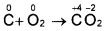Oxidizing agent – O
Reducing agent-C

Question 46.
Analyze the oxidation number and note down the oxidizing agent and reducing agent in the following reaction.a) Oxidation number of which atom is increased?
b) The oxidized atom is
c) Oxidation number of which atom is decreased?
d) The reduced atom is
e) Oxidizing agent
f) Reducing agent
a) Na
b) Na
c) Cl
d) Cl
e) Na
f) Cl

Question 47.
Analyze the following equation fill-up the blanks.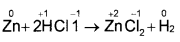Question 48.
The oxidation number of zinc increases/decreases from … to…
0 to +2

Question 49.
The oxidized atom is…
Zn

Question 50.
The oxidation number of hydrogen increases/decreases from …. to
+1 to 0

Question 51.
The reduced atom is …
H
Here HCI is the oxidizing agent and Zn is the reducing agent. „

Question 52.
How do you determine the oxidation number of sulfur in H2 SO4?
Oxidation state of hydrogen = +1
Oxidation state of oxygen = -2
Let the oxidation state of sulphur be ‘x’ We know the sum of oxidation states of all atoms in a compound is zero. Therefore,
[2×(+1)] + x + (4x – 2) = 0
(+2) + x + (-8) = 0
x – 6 = 0
x = +6

Question 53.
Find the oxidation number of Mn in KMnO4 (oxidation number of K is +1, oxidation number of O is -2)
Oxidation state of potassium = +1
Oxidation state of oxygen = -2
Let the oxidation state of Mn be ‘x’.
1 x (+1) + x + 4(-2) = 0
(+1) + x + (-8) = 0
x – 7 =0
x = +7

Question 54.
Find the oxidation number of Mn in MnO2, Mn2O3 and Mn2O7
MnO2
Mn + 2x -2 = 0
Mn + 4 = 0
Mn = +4

Mn2O3
2Mn + 2x -2 = 0
2Mn + -4 = 0
2 Mn = +4
Mn = +2

Mn2O7
2Mn + -2×7 = 0
2Mn + -14 = 0
2Mn = +14
Mn = +7

Question 55.
What is Redox reaction?
The process of oxidation and reduction take place simultaneously. Hence these two reactions together are known as redox reaction.
Examples:
H2 + Cl2 → 2HCl
Mg + F2 → MgF2
2Na + Cl2 → 2NaCl

Question 56.
What are the methods usually adopted to make firewood burn faster? ‘
1. Provide more air
2. Split up into small pieces
3. Make firewood dryQuestion 57.
Describe an experiment to prove that nature of the reactants affects the rate of chemical reaction.
Materials required for the experiment.
Zn, Mg, dil. HCl arid test tubes.
Procedure:
Take equal volume of dil. HCl in two test tubes. Add Zn to one and Mg of same mass to the other. Hydrogen gas is produced in both the test tubes. Rate of reaction is faster in the test tube containing Mg.

Question 58.
Write an experiment to prove that concentration of reactants affect the rate of reactions.
Materials required: Mg, dil. HCl, Con. HCl and test tubes.
Procedure: Take magnesium ribbons of equal mass in two test tubes. Add concentrated HCl to one test tube and dilute HCl to the other in equal volume

Question 59.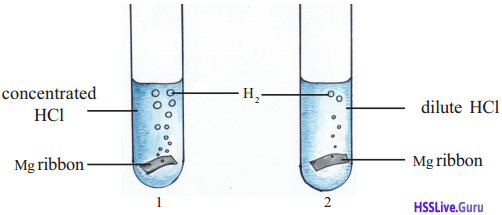Test tube 1: ……………
Test tube 2: …………..
Test tube 1: Reaction is faster in con. HCl
Test tube 2: Reaction rate is slow

Question 60.
Why rate of reaction increases when concentration increases?
As the concentration of reactants increases, the number of molecules per unit volume and the number of effective collisions increase. Consequently the rate of reaction increases.

Question 61.
What is the relation between rate of reaction and surface area? Write an experiment to prove it.
Take equal volume of dil. HCI in two beakers. Add a small piece of marble into one and marble powder of equal mass into the other. Reaction rate is greater when marble powder is used. Rate of reaction increases when surface area increases

Question 62.Is there any difference in the rate of reaction in the two beakers?
Reaction rate is greater when powdered marble is used.

Question 63.
What about the concentration of acid in both the reactions?
Same

Question 64.
Is there any difference in the mass of the marble?
No

Question 65.
What about the surface area of marble?
Different

Question 66.
In which case is the chance for greater number of acid molecules to get in contact with marble, in a given time?
If marble is powdered

Question 67.
What is the change in the rate of collision when surface area increases?
When surface area increases rate of reaction also increases.

Question 68.
What will happen to the rate of this reaction if marble is further crushed or powdered?
Will increase

Question 69.
Why rate of reaction increases when surface area increases?
When solids are made into small pieces or powder, their surface area increases. As a result the number of molecules undergoing effective collision also increases. Hence the rate of reaction increases.

Question 70.
Write an experiment to prove the relation between temperature and rate of reaction.
Materials required: Sodium thiosulphate, hydrochloric acid, water, boiling tube, spirit lamp. Procedure: Prepare dilute solution of sodium thiosulphate in a beaker. Take equal volumes of this solution in two boiling tubes. Heat one boiling tube for some time. Add dilute hydrochloric acid in equal amounts in both the boiling tubes.
Observation: Reaction is faster in heated test tube.

Question 71.
In which of the boiling tubes is the precipitate formed faster?
In heated test tube

Question 72.
What is the color of the precipitate formed?
Pale yellow

Question 73.
What is Threshold Energy?
To take part in a chemical reaction, molecules should attain certain minimum kinetic energy. This energy is called threshold energy.Question 74.
Write an experiment to prove the influence of catalysts in a chemical reaction.
Take some hydrogen peroxide solution in a test tube. Show a glowing incense stick into the test tube.
What is the observation? Is there any difference occurring in the way in which the incense stick bums? No . ‘
Now add some manganese dioxide (MnO2) into the test tube. Again show the glowing incense stick. Observation:
Glowing incense stick flares up now.

It indicates that when manganese dioxide is added, the rate of reaction increases and oxygen is formed faster. Filter the solution using a filter paper when the reaction is completed.

The substance remaining in the filter paper is manganese dioxide itself When examined carefully it becomes clear that there is no change in its amount or property. The presence of manganese dioxide has increased the rate of the reaction. Manganese dioxide acts as a catalyst in this reaction.

Question 75.
What are catalysts?
Catalysts are substances which alter the rate of chemical reactions without themselves undergoing any permanent chemical change.

### Redox Reactions and Rate of Chemical Reactions Additional Questions and Answers

Magnesium combines with chlorine to form magnesium chloride. The equation is given below
Mg + Cl2 → MgCl2

Question 1.
Write down the electronic configuration of magnesium and chlorine
Mg – 2, 8, 2
Cl – 2, 8,7

Question 2.
How many electrons are donated by magnesium? Write the chemical equation for this process
2, Mg → Mg2+ +2e

Question 3.
How many electrons are accepted by chlorine?
1, charge – ve

Question 4.
Write the chemical equation.
Cl + 1e → cl

Question 5.
Explain oxidation, reduction, oxidizing agent and reducing agent?

• Oxidation is the process by which the removal of electrons.
• Reduction is the process by which the addition of electrons.
• In the above process, magnesium reduces chlorine by donating electrons to it hence magnesium is the reducing agent. In this process since chlorine oxidizes magnesium by accepting electrons it is considered as the oxidizing agent.

Question 6.
From the given chemical equation identify the oxidizing agent and the reducing agent by writing the chemical equation of oxidation and reduction
(i) Mg + F2 → MgF2
(ii) 2Na + Cl2 → 2NaCl
(i) Mg → Mg2+ + 2e (Oxidation)
F + 1e → F (Reduction)
Here magnesium is the reducing agent and fluorine is the oxidising agent.
(ii) Na → Na+ + 1e (Oxidation)
Cl + 1e → Cl (Reduction)
Here sodium is the reducing agent and chlorine is the oxidizing agent.

Question 7.In the formation of hydrogen chloride does the oxidation number of hydrogen increase or decrease?
The oxidation number of hydrogen increases from 0 to +1

Question 8.
Whether the oxidation number of chlorine decrease or increase?
The oxidation number of chlorine decreases from 0 to — 1.

Question 9.
Based on oxidation number which is the oxidizing agent and reducing agent?
H2 is the reducing agent and Cl2 is the oxidizing agent.

Question 10.
What is meant by Redox reaction?
A process in which the increase in oxidation number is the oxidation and decrease in oxidation number is the reduction. A process in which both oxidation and reduction takes place is the redox reaction. The molecule in which the atom whose oxidation number decreases during the process is the oxidizing agent and that in which oxidation number of an atom increase is the reducing agent.

Question 11.
In the following chemical reactions which is the oxidizing agent which is the reducing agent? Analyze the oxidation number and find out?Reducing agent – C
Oxidising agent – Cl2

Question 12.
Given below is the equation of the reaction between zinc and hydrochloric acid also indicating the oxidation number.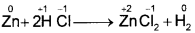Analyze and find the following:
In this process, oxidation number of zinc increases from 0 to +2.
Oxidation number of hydrogen decrease from +1 to 0 The reducing agent is zinc and oxidising agent is HCl.

Question 13.
Calculate the oxidation number of‘S’ in H2 SO4?
Oxidation number of hydrogen = +1
Oxidation number of oxygen = -2
Let the oxidation number of ‘S’ be x
The sum of the oxidation numbers of all the Elements in a compound is zero,
Hence
[2x (+1] + x + 4 x (-2] = 0
2 + x – 8 = 0
x = +8 – 2
x = +6
ie., oxidation number of ‘S’ in H2 SO4 is +6.Question 14.
Find the oxidation number of the ions in ionic compound?
The charge of ions in ionic compound is the oxidation number. Eg. In FeCl2 the oxidation number of Fe is +2 and FeCl3 is +3.

Let Us Assess

Some chemical equations are given below.
C + O2 → CO2
CH4 + 2O2 → CO2 + 2H2O
N2 + O2 → NO
CaCO2 → CaO + CO2
H2 + l2 → Hl
Fe + HCl → FeCl2 + H2
CO2 + C → CO
a) Which of these are balanced equations?
b) Balance the unbalanced equations.
c) Which among these are redox reactions?
a) C + O2 → CO2
CH4 + 2O2 → CO2 +2 H2O
CaCO3 → CaO +CO2
b) N2 + O2 → 2NO
H2 + l2 → 2Hl
Fe + 2HCl → FeCl2 + H2
CO2 + C2CO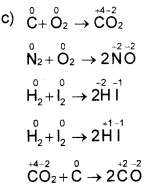Question 2.
The chemical reaction between marble and dilute HCl is given.
CaCO3 + 2HCl → CaCl2 + H2O +CO2
a) Which gas is formed here? How can you identify this gas?
b) Suggest any two ways you would choose to increase the rate of this chemical reaction. Explain the reason.
a) Carbon dioxide (CO2). Show a burning splinter in the gas. It will get extinguished.
or
Show a glass rod dipped in clear lime water into the gas. The lime water will turn milky
b) 1) Use powdered marble
2) Use concentrated HCl
3) Heat the mixture.

Question 3.
Sulfur pieces do not react with cold concentrated nitric acid. But sulfur powder reacts.
a) Explain the reason why the rate of chemical reaction is increased here?
b) Suppose you want to increase the rate of reaction again. Which way you would choose? Give reason.
a) When surface area increases rate of effective collision increases. So the rate of reaction increases.
b) Heat the mixture.
When temperature increases more molecules will get threshold energy. So rate of effective collision and rate of reaction increases.

Question 4.
Small amounts of phosphoric acid is usually added to hydrogen peroxide to prevent its decomposition?
a) What is the function of phosphoric acid here?
b) By which name are these type of substances known?
c) Which substance would you add to increase the rate of decomposition of hydrogen peroxide?
a) Slow down the rate of decomposition of hydrogen peroxide
b) Negative catalyst
c) Manganese dioxide

Extended Activities

Question 1.
Find the oxidation number of the elements which are underlined in the compounds given below. Among these find out the elements which show variable oxidation numbers.
MnO2, Mn2O7, K2Cr2O7, KCrO3, MnCl2, MgO, MgCl2, Al2O3,AlCl3
(Hint. Oxidation number 0 = -2, Cl = -1, K = +1)
MnO2 Let x be the oxidation number of Mn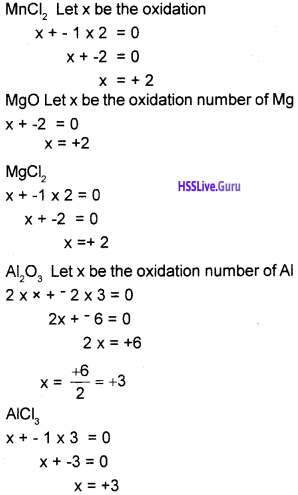Question 2.
Some apparatus and chemicals are given.
Zn, Mg, dilute HCl, CaCO3, test tube, water.
a) Design an experiment to prove that the nature of reactants can influence the rate of reaction.
b) Write the equations for the chemical reactions.
c) Write the expression for the rate of the reaction.
a) Take equal volume of dil HCl in two test tubes. Add a piece of Mg m to one of them. Add a piece of Zn of equal mass to the other.
Observation:- Mg reacts faster with dil. HCl than Zn. This is due to the difference in the chemical nature of Mg and Zn.
b) Mg + 2HCl → MgCl2 +H2
Zn + 2HCl → ZnCl2 + H2
c) Rate of a chemical reaction $$=\frac{\text { amount of reactants used up }}{\text { time }}$$
= $$\frac{\text { amount of product formed }}{\text { time }}$$Question 3.
The experiments conducted by two students are given below.
Experiment 1: 2 mL of sodium thiosulphate solution is taken in a test tube, heated and to it 2 mL of HG solution is added.
Experiment 2:2 mL of sodium thiosulphate solution is taken in a test tube and to it 2 mL of HC1 solution is added.
a) In which experiment is the precipitate formed quickly? Justify your answer.
b) Write the balanced equation for the reaction.